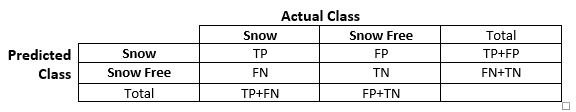# Classification of Imbalanced Data

Recently, I was asked to come up with a machine learning approach to detect snow in images. What if I told you, I could come up with a classifier on the spot that classifies, I would say, at least 85% of the given images correctly?

## Skewed Data

Let’s first think about the Classification of Imbalanced Data problem.

We have a lot of images, lets say for the past year. Each image has snow in it or not. In most European regions, snow is highly less likely than snow free conditions.

So what does that tell you for your data? It is skewed!

Your data has the same percentual distribution between snow-free and snow data. Therefore, 80-90% of our images most likely do not depict snow.

So, can you come up with a classifier with 80-90% accuracy? It is quite easy:

``return NO_SNOW;``

Your precise classifier always return NO_SNOW without even looking at your input data!

Of course, we are not satisfied with this result. Interestingly enough, most often we are more interested in the minority than the majority class (for example in fraud detection or anamoly detection in general).

In the following, I give a short introduction to the skewed data problem, I lay out approaches how you can come up with a better, more intelligent classifier, and what performance measures I would recommend to evaluate your classifier in the presence of skewed data.

## Forecast Terminology

A data set is called skewed or imbalanced when one of the classes highly dominates the others.

Congestion detection is a classic example of imbalanced data in real-world applications.

We can assume that free-flowing traffic conditions have much higher probability than congested conditions (see Figure 1).

More general, the task is to map the input variables (feature vector) to a specific class.

Algorithms for pattern recognition are usually trained from labelled data where the individual observations are correctly classified (supervised learning).

In our snow example, the dominating majority (negative) class is represented by the data points representing snow-free conditions.

The minority (positive) class is represented by rare instances of data points representing snow.

The problem gets even worst when you split for data into train, test, and validation set. Thereby you might end up with a training set only containing examples of the majority class.

Machine learning algorithms are likely to fail to build a good model for skewed data.

The resulting lack of training instances for the minority class makes the learning process more difficult.

I once had the case that my trained Support Vector Machine exactly learned what our dumb model from before what do. It just always predicted the majority class.

## Forecast Evaluation Measures

In terms of classification, you typically evaluate the True and False Positives (TP/FP), as well as the True and False Negatives (TN/FN), depicted in a Confusion Matrix (see Figure 2).

Here, we would say a TP is when the classifier classifies a condition as snow and the image shows snow.

Again, imbalanced data means that data points belonging to TP (snow) are much less likely than FP (no snow).Fig. 2 Confusion matrix reporting the number of true positives (TP), false positives (FP), false negatives (FN), and true negatives (TN).

These four variables are then used to calculate other measures, such as Precision P, Accuracy A, Recall R, Specifity S, and F-measure F.

`P = TP / (TP+FP)`
`A = (TP+TN) / N`
`R = TP / (TP+FN)`
`SP = TN / (FP+TN)`
`F = 2PR / (P+R)`

However, the consideration of only a single metric can be deceiving.

A classifier who simply classifies all situations as snow-free achieves high Accuracy as the number of TN will be rather high.

High Recall can easily be achieved by classifying all situations as snow.

On the other hand, an algorithm who predicts few or no snow may result in high Precision since the number of FP is minimised.

The F-measure addresses this problem and considers both Recall R and Precision P.

Consequently, you should not just consider one or two measures but all in combination to cover all aspects.

In terms of very imbalanced data, a good classifier is depicted by high values for Precision and Recall, and consequently by a high value of the F-measure.

## Remove Skewness

Another possibility to remove the skewness from the data is to add examples to the minority class.

That means we synthetically create data points which would fall into the less represented class. Thereby, we fill it up until we have a more less even distribution.

In our snow example, we would have to create images with snow on them or congested data points in term of congestion detection. To can synthesize new samples from the under-represented class  by using an algorithm like SMOTE.

Another approach is to adapt the class weights or parameter costs for the different classes, e.g. to penalize the classifier for false positives more then for false negatives.

Besides that, there exist some methods which are design specifically to work well with imbalanced data.

Ensemble Learning seems to be one of the solution where you do not rely solely on the outcome of one classifier but combine the results of severals.

Each method classifies an input based on his internal model and their independent votes are combined into the final classification result.

## References

A must read for every forecast practioneer is “Forecasting: Principles and Practices” by J. Hyndman https://otexts.org/fpp2/

Learning from Inbalanced Data: https://ieeexplore.ieee.org/document/5128907

Learning Classifier Systems for Road Traffic Congestion Detection: http://www.scitepress.org/DigitalLibrary/PublicationsDetail.aspx?ID=y680gmUuBiI=&t=1